## Posts

### Conjugate beam Problem no:6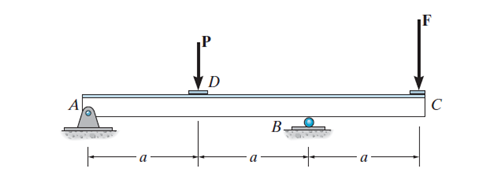Example 5: determine the force F, so the displacement at C will be zero assuming EI constant over the beam length? Figure 1 The first step is to draw the M/EI diagram for the real beam. The conjugate beam will be loaded with M/EI diagram for the real beam. The pin at A will stay the same for the conjugate beam while the internal roller at B will change to an internal pin for the conjugate beam. The free end at point C will become a fixed end. So the conjugate beam will be as shown in figure no:2.  Figure 2 To draw M/EI, we need to determine A and B's reaction for the real beam. Figure 3 So the shear and moment diagram will be as shown in figure no:4. Figure 4 Now, we will take section B-C. to determine FB using the equilibrium equation(MC=0). Figure 5 Now, we will take section A-B. to determine FB using the equilibrium equation. The FB computed from section A-B will be equaled to Fb calculated from section B-C to determine F related to P. To calculate a1 and a2, we will use the t

### Nominal maximum size of coarse aggregate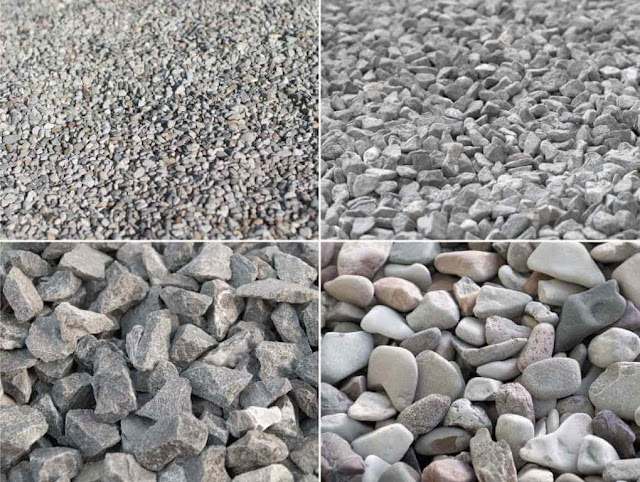Concrete is a widely used material. Reinforced concrete structures can be found everywhere. The main reason behind the popularity of reinforced concrete structure is the high strength and the long life span of these structures. Concrete and steel are combined to produce reinforced concrete. Concrete is good at compression while steel good at tension. Concrete and steel form a perfect combination to resist loads imposed on the structure. Concrete is a composite material composed of cement, coarse aggregate, fine aggregate, and water. Admixtures can be used to enhance concrete properties. A concrete mix consists of almost 65% aggregates. Aggregates are classified into fine and coarse aggregates. Since the aggregates form the majority of a concrete mix. The quality of aggregates can greatly affect the strength and durability of concrete. Aggregates can be natural or crushed aggregates. Used aggregates should be strong, hard, and should not contain a large amount of organic matter or harmf

### Conjugate beam Problem no:5Example 5: determine the displacement at C and the slope at point B for the beam shown in figure no: 1 considering EI a constant? Figure 1   The support at A for this beam will allow the rotation but no movement, so it is considered a pin, and it will stay the same in the conjugate beam. While the support at B will allow the movement in horizontal direction and rotation, it will be similar to the roller, and it will stay the same in the conjugate beam. So the real beam will be as in figure no:2. And the conjugate beam will be as shown in figure no:3. Figure 2 Figure 3 To determine the displacement at point C and the slope at B, we need to determine the moment at point C and the shear at point B in the conjugate beam. The conjugate beam will be loaded with M/EI of the real beam, so we need to draw M/EI for the real beam. To draw the M/EI diagram, we need to determine the reaction at point A and point B. From the symmetry we can know that the reaction FA=FB=P. so the shear moment diagram

### Bar Bending schedule (BBS)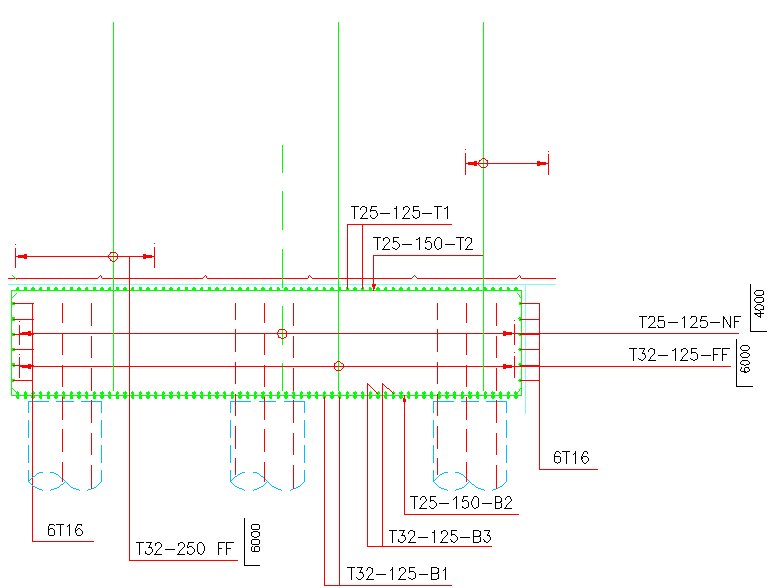The bar  bending schedule is a table that contains the details of reinforcement for a specific structural member such as footing, column, slab, and others. The bar bending schedule will contain the bars' dimensions to be used, the shape of the bars, the size of bars, bar mark, number of bars, and the weight of bars. BBS will be used to determine the weight of reinforcement required for a specific structural member.  there are various advantages of bar bending schedule such as: estimating the quantity of reinforcement for each structural member. So we can estimate the amount of reinforcement required for a construction project.  BBS will help in reducing the losses of steel by procuring the precise quantity of different rebar diameter.  BBS will reduce the wastage of reinforcement by providing the engineers with the exact dimension and shape of rebars. Knowing the exact dimension and shape will reduce the time of cutting and bending rebars.  BBS will reduce the effort at the time o

### Conjugate beam Problem no:4 (Beam with internal pin, roller and point load )Example no:4: find the displacement at point C and the slope at point B for the beam shown in figure no:1. Figure 1 The conjugate beam will be, as shown in figure no:2. The pin and roller at A and B will become internal pin. The free ends will become fixed, as shown in figure no:2. We need to determine the M/EI diagram for the real beam, which will be the load in the conjugate beam, to determine the displacement at point c and slope at point B. Figure 2 To draw the moment diagram for the beam, we need to determine the reaction at point A and B, then draw the shear diagram and the moment diagram. Using the equilibrium equations: Figure 3 The shear and moment diagram will be, as shown in figure no:4. Figure 4 The conjugate beam will be loaded, as shown in figure no:5. Figure 5 To determine the displacement at point C, we need to determine the moment in the conjugate beam at point C. to determine the moment at point C, we will take section A-B to determine the force at B. Figure 6 Now we

### Binding wire for steel reinforcement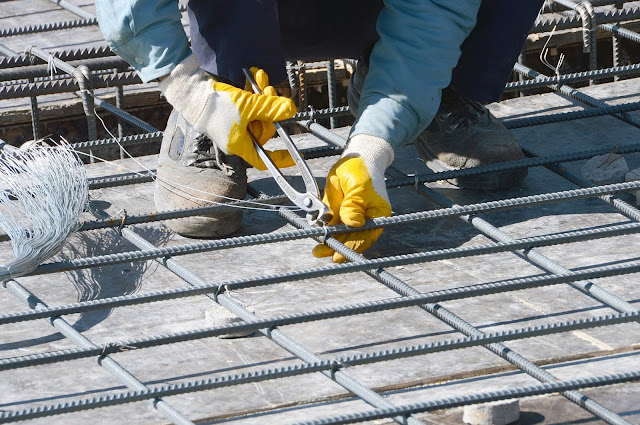Binding wires are used to tie steel bars together. These wires are playing a significant role in maintaining the reinforcement stability and rigidity. Wires are used to tie the steel bars at intersections points. By tying the steel bars together, we ensure that the steel bars will not move from their locations during construction or during the time of concreting. In slabs, binding wires are used to tie longitudinal and transverse bars together. In columns, it is used to tie vertical bars with stirrups.   Figure 1 There are various types of binding wires such as  Black annealed baling wire, Stainless Steel Binding Wire, and PVC coated binding wires.  Black annealed baling wire used to tie black steel. The popular size for black wire ranges from 16 to 22 gauges. Stainless steel binding wires are used to bind stainless steel reinforcement. Stainless steel reinforcement is used in a harsh environment where the black steel gets rusted quickly. Therefore, stainless steel reinforcement is u

### Conjugate beam Problem no:3 (Determining the distance a)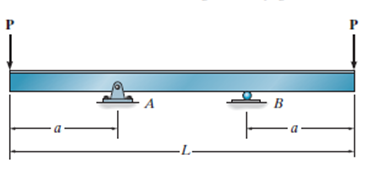Determine the distance "a" so the displacement at the edge of the beam equals the displacement at the center of the beam? Figure 1 The conjugate beam will be, as shown in figure no:2. The pin and roller at A and B will become internal pin. The free ends will become fixed, as shown in figure no:2. We need to determine the M/EI diagram for the real beam, which will be the load in the conjugate beam, so we can determine the distance “a” that will cause an equal displacement at the end and center of the beam.  Figure 2 Using the equilibrium equations, we will determine the reaction at point A and point B. Figure 3 After determining the reactions at support A and B, we can now draw the shear and moment diagram. The M/EI will be the load on the conjugate beam as shown in figure no:4 and 5. Figure 4 Figure 5 Now we need to equal the moment at the end beam and the center of the beam. we assume the distance between A and B equals L1 and L1=L-2*a. To simplify the solution, we will ta